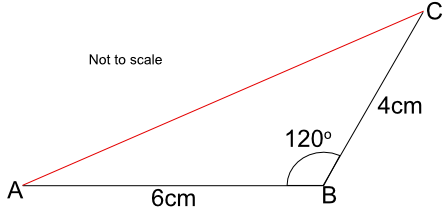Drawing Diagrams from Descriptions

## Drawing Diagrams from Descriptions

A ruler is used for measuring distances and drawing straight lines.

A protractor is used for measuring angles. Make sure that the centre of the protractor (the cross-hairs) is on the point of the angle, and that one of the zero lines lies along one of the angle lines.

A pair of compasses are for drawing radii. The radii may be for a circle of a given radius, or may be an arc.

Set squares - the triangular shapes from a geometry set - are used on engineering drawings to draw parallel lines. Both set squares will have a 90º angle: one set square will have angles of 45º/45º, and the other 30º/60º.

Dividers - which look like a pair of compasses but with two point and no pencil - are used to measure distances.

## Example 1

Two lines AB and BC intersect at B with an angle of 120º as shown in the diagram. Line AB is 6cm long, and line BC is 4cm long. By accurately drawing the lines, determine the length of the line AC.Draw the line AB 6cm long.

At point B, measure an angle of 120º.

Draw a line from B through the measured angle.

Measure 4cm along this line and mark 4cm.

Measure the line AC.

You should be within 1mm of the correct answer.

## Example 2

By drawing the diagram, measure the angle ACB.Draw the line AB.

Using a pair of compasses, draw arcs 4cm from point A and from point B, such that the arcs intersect.

Join the intersection of the arcs to point A, and to point B.

Measure the angle with a protractor.